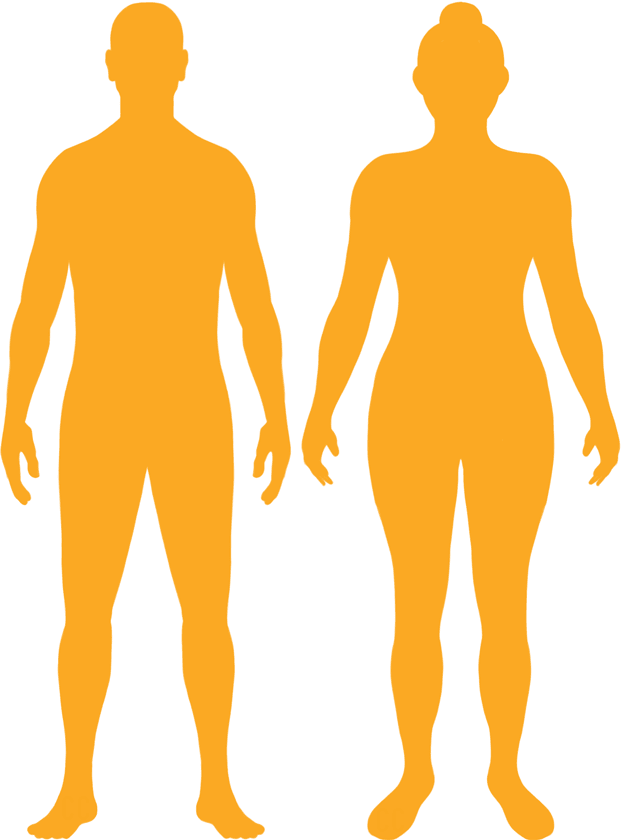# BMI Calculator

Result for 5'1" and 148 lbs.
Change Here.27.96

### Overweight BMI

At 5'1" and 148 Pounds your BMI is 27.96

### BMI Scale

 Under Normal Over Obese
-16 lbs
to reach a
normal BMI classification.
##### Normal BMI Ends:
132.3 lbs
+11 lbs
until you reach an obese BMI classification.
158.8 lbs

### BMI Grading Table2 for Height 5'1"

 Weight Weight Class Below 97.9 lbs Underweight 97.9 lbs - 132.3 lbs Normal Weight 132.3 lbs - 158.8 lbs Overweight 158.8 lbs and Above Obese

### Weight Loss

If you were seeking a normal BMI weight of 132.3lbs, and you lost two pounds a week4, then you could reach a normal BMI in
8 Weeks

### Overweight BMI Silhouette### Ideal Weight for a 5 foot 1 Male or Female2

The ideal weight range using the BMI for a male or female with a height of 5'1" is

### Between

97.9 lbs

and
132.3 lbs
How to Calculate BMI3 for 5'1" and 148 lbs
(5 x 12) + 1 = 61
(148 / (61 x 61)) x 703
= 27.961300725611
5 = foot | 1 = inch | 148 = weight lbs

### Conversion

• 5 foot 1 = 61 inches
• 5 foot 1 = 154.94cm
• 148 pounds = 10 stone 8 pounds
• 148 pounds = 67.13 kg
• 148 pounds = 67131.67 grams

### 5'1" and 148 lbs Summary

What is the BMI for a 5'1" and 148 lbs female? 27.96 BMI, Overweight.
What is the BMI for a 5'1" and 148 lbs male? 27.96 BMI, Overweight.
What is the ideal weight for a 5'1" female? Between: 97.9lbs and 132.3lbs
What is the ideal weight for a 5'1" male? Between: 97.9lbs and 132.3lbs
If I am 5ft 1in and weigh 148 lbs, is that a good weight for my height? Under the BMI classification, 148 lbs is classed as being Overweight.